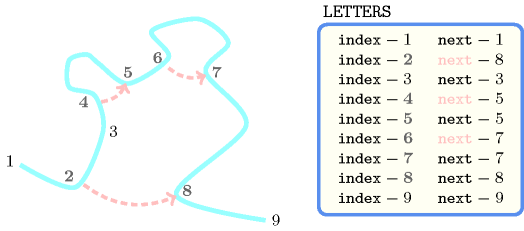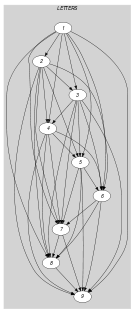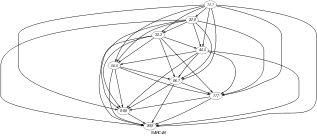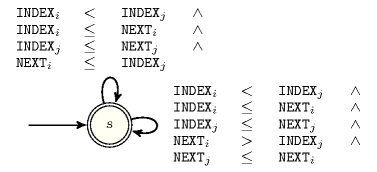## 5.343. sequence_folding

Origin

J. Pearson

Constraint

$\mathrm{𝚜𝚎𝚚𝚞𝚎𝚗𝚌𝚎}_\mathrm{𝚏𝚘𝚕𝚍𝚒𝚗𝚐}\left(\mathrm{𝙻𝙴𝚃𝚃𝙴𝚁𝚂}\right)$

Argument
 $\mathrm{𝙻𝙴𝚃𝚃𝙴𝚁𝚂}$ $\mathrm{𝚌𝚘𝚕𝚕𝚎𝚌𝚝𝚒𝚘𝚗}\left(\mathrm{𝚒𝚗𝚍𝚎𝚡}-\mathrm{𝚒𝚗𝚝},\mathrm{𝚗𝚎𝚡𝚝}-\mathrm{𝚍𝚟𝚊𝚛}\right)$
Restrictions
 $|\mathrm{𝙻𝙴𝚃𝚃𝙴𝚁𝚂}|\ge 1$ $\mathrm{𝚛𝚎𝚚𝚞𝚒𝚛𝚎𝚍}$$\left(\mathrm{𝙻𝙴𝚃𝚃𝙴𝚁𝚂},\left[\mathrm{𝚒𝚗𝚍𝚎𝚡},\mathrm{𝚗𝚎𝚡𝚝}\right]\right)$ $\mathrm{𝙻𝙴𝚃𝚃𝙴𝚁𝚂}.\mathrm{𝚒𝚗𝚍𝚎𝚡}\ge 1$ $\mathrm{𝙻𝙴𝚃𝚃𝙴𝚁𝚂}.\mathrm{𝚒𝚗𝚍𝚎𝚡}\le |\mathrm{𝙻𝙴𝚃𝚃𝙴𝚁𝚂}|$ $\mathrm{𝚒𝚗𝚌𝚛𝚎𝚊𝚜𝚒𝚗𝚐}_\mathrm{𝚜𝚎𝚚}$$\left(\mathrm{𝙻𝙴𝚃𝚃𝙴𝚁𝚂},\mathrm{𝚒𝚗𝚍𝚎𝚡}\right)$ $\mathrm{𝙻𝙴𝚃𝚃𝙴𝚁𝚂}.\mathrm{𝚗𝚎𝚡𝚝}\ge 1$ $\mathrm{𝙻𝙴𝚃𝚃𝙴𝚁𝚂}.\mathrm{𝚗𝚎𝚡𝚝}\le |\mathrm{𝙻𝙴𝚃𝚃𝙴𝚁𝚂}|$
Purpose

Express the fact that a sequence is folded in a way that no crossing occurs. A sequence is modelled by a collection of letters. For each letter ${l}_{1}$ of a sequence, we indicate the next letter ${l}_{2}$ located after ${l}_{1}$ that is directly in contact with ${l}_{1}$ (${l}_{1}$ itself if such a letter does not exist).

Example
$\left(\begin{array}{c}〈\begin{array}{cc}\mathrm{𝚒𝚗𝚍𝚎𝚡}-1\hfill & \mathrm{𝚗𝚎𝚡𝚝}-1,\hfill \\ \mathrm{𝚒𝚗𝚍𝚎𝚡}-2\hfill & \mathrm{𝚗𝚎𝚡𝚝}-8,\hfill \\ \mathrm{𝚒𝚗𝚍𝚎𝚡}-3\hfill & \mathrm{𝚗𝚎𝚡𝚝}-3,\hfill \\ \mathrm{𝚒𝚗𝚍𝚎𝚡}-4\hfill & \mathrm{𝚗𝚎𝚡𝚝}-5,\hfill \\ \mathrm{𝚒𝚗𝚍𝚎𝚡}-5\hfill & \mathrm{𝚗𝚎𝚡𝚝}-5,\hfill \\ \mathrm{𝚒𝚗𝚍𝚎𝚡}-6\hfill & \mathrm{𝚗𝚎𝚡𝚝}-7,\hfill \\ \mathrm{𝚒𝚗𝚍𝚎𝚡}-7\hfill & \mathrm{𝚗𝚎𝚡𝚝}-7,\hfill \\ \mathrm{𝚒𝚗𝚍𝚎𝚡}-8\hfill & \mathrm{𝚗𝚎𝚡𝚝}-8,\hfill \\ \mathrm{𝚒𝚗𝚍𝚎𝚡}-9\hfill & \mathrm{𝚗𝚎𝚡𝚝}-9\hfill \end{array}〉\hfill \end{array}\right)$

Figure 5.343.1 gives the folded sequence associated with the previous example. Each number represents the index of an item. The $\mathrm{𝚜𝚎𝚚𝚞𝚎𝚗𝚌𝚎}_\mathrm{𝚏𝚘𝚕𝚍𝚒𝚗𝚐}$ constraint holds since no crossing occurs.

##### Figure 5.343.1. Folded sequence (in blue) of the Example slot: links from a letter to a distinct letter are represented by a dashed arc, while self-loops are not drawnTypical
 $|\mathrm{𝙻𝙴𝚃𝚃𝙴𝚁𝚂}|>2$ $\mathrm{𝚛𝚊𝚗𝚐𝚎}$$\left(\mathrm{𝙻𝙴𝚃𝚃𝙴𝚁𝚂}.\mathrm{𝚗𝚎𝚡𝚝}\right)>1$
Usage

Keywords
Arc input(s)

$\mathrm{𝙻𝙴𝚃𝚃𝙴𝚁𝚂}$

Arc generator
$\mathrm{𝑆𝐸𝐿𝐹}$$↦\mathrm{𝚌𝚘𝚕𝚕𝚎𝚌𝚝𝚒𝚘𝚗}\left(\mathrm{𝚕𝚎𝚝𝚝𝚎𝚛𝚜}\right)$

Arc arity
Arc constraint(s)
$\mathrm{𝚕𝚎𝚝𝚝𝚎𝚛𝚜}.\mathrm{𝚗𝚎𝚡𝚝}\ge \mathrm{𝚕𝚎𝚝𝚝𝚎𝚛𝚜}.\mathrm{𝚒𝚗𝚍𝚎𝚡}$
Graph property(ies)
$\mathrm{𝐍𝐀𝐑𝐂}$$=|\mathrm{𝙻𝙴𝚃𝚃𝙴𝚁𝚂}|$

Arc input(s)

$\mathrm{𝙻𝙴𝚃𝚃𝙴𝚁𝚂}$

Arc generator
$\mathrm{𝐶𝐿𝐼𝑄𝑈𝐸}$$\left(<\right)↦\mathrm{𝚌𝚘𝚕𝚕𝚎𝚌𝚝𝚒𝚘𝚗}\left(\mathrm{𝚕𝚎𝚝𝚝𝚎𝚛𝚜}\mathtt{1},\mathrm{𝚕𝚎𝚝𝚝𝚎𝚛𝚜}\mathtt{2}\right)$

Arc arity
Arc constraint(s)
$\bigvee \left(\begin{array}{c}\mathrm{𝚕𝚎𝚝𝚝𝚎𝚛𝚜}\mathtt{2}.\mathrm{𝚒𝚗𝚍𝚎𝚡}\ge \mathrm{𝚕𝚎𝚝𝚝𝚎𝚛𝚜}\mathtt{1}.\mathrm{𝚗𝚎𝚡𝚝},\hfill \\ \mathrm{𝚕𝚎𝚝𝚝𝚎𝚛𝚜}\mathtt{2}.\mathrm{𝚗𝚎𝚡𝚝}\le \mathrm{𝚕𝚎𝚝𝚝𝚎𝚛𝚜}\mathtt{1}.\mathrm{𝚗𝚎𝚡𝚝}\hfill \end{array}\right)$
Graph property(ies)
$\mathrm{𝐍𝐀𝐑𝐂}$$=|\mathrm{𝙻𝙴𝚃𝚃𝙴𝚁𝚂}|*\left(|\mathrm{𝙻𝙴𝚃𝚃𝙴𝚁𝚂}|-1\right)/2$

Graph model

Parts (A) and (B) of Figure 5.343.2 respectively show the initial and final graph associated with the Example slot. Since we use the $\mathrm{𝐍𝐀𝐑𝐂}$ graph property, the arcs of the final graph are stressed in bold.

##### Figure 5.343.2. Initial and final graph of the $\mathrm{𝚜𝚎𝚚𝚞𝚎𝚗𝚌𝚎}_\mathrm{𝚏𝚘𝚕𝚍𝚒𝚗𝚐}$ constraint(a) (b)
Signature

Consider the first graph constraint. Since we use the $\mathrm{𝑆𝐸𝐿𝐹}$ arc generator on the $\mathrm{𝙻𝙴𝚃𝚃𝙴𝚁𝚂}$ collection the maximum number of arcs of the final graph is equal to $|\mathrm{𝙻𝙴𝚃𝚃𝙴𝚁𝚂}|$. Therefore we can rewrite the graph property $\mathrm{𝐍𝐀𝐑𝐂}=|\mathrm{𝙻𝙴𝚃𝚃𝙴𝚁𝚂}|$ to $\mathrm{𝐍𝐀𝐑𝐂}\ge |\mathrm{𝙻𝙴𝚃𝚃𝙴𝚁𝚂}|$ and simplify $\underline{\overline{\mathrm{𝐍𝐀𝐑𝐂}}}$ to $\overline{\mathrm{𝐍𝐀𝐑𝐂}}$.

Consider now the second graph constraint. Since we use the $\mathrm{𝐶𝐿𝐼𝑄𝑈𝐸}\left(<\right)$ arc generator on the $\mathrm{𝙻𝙴𝚃𝚃𝙴𝚁𝚂}$ collection the maximum number of arcs of the final graph is equal to $|\mathrm{𝙻𝙴𝚃𝚃𝙴𝚁𝚂}|·\left(|\mathrm{𝙻𝙴𝚃𝚃𝙴𝚁𝚂}|-1\right)/2$. Therefore we can rewrite the graph property $\mathrm{𝐍𝐀𝐑𝐂}=|\mathrm{𝙻𝙴𝚃𝚃𝙴𝚁𝚂}|·\left(|\mathrm{𝙻𝙴𝚃𝚃𝙴𝚁𝚂}|-1\right)/2$ to $\mathrm{𝐍𝐀𝐑𝐂}\ge |\mathrm{𝙻𝙴𝚃𝚃𝙴𝚁𝚂}|·\left(|\mathrm{𝙻𝙴𝚃𝚃𝙴𝚁𝚂}|-1\right)/2$ and simplify $\underline{\overline{\mathrm{𝐍𝐀𝐑𝐂}}}$ to $\overline{\mathrm{𝐍𝐀𝐑𝐂}}$.

Automaton

Figure 5.343.3 depicts the automaton associated with the $\mathrm{𝚜𝚎𝚚𝚞𝚎𝚗𝚌𝚎}_\mathrm{𝚏𝚘𝚕𝚍𝚒𝚗𝚐}$ constraint. Consider the ${i}^{th}$ and the ${j}^{th}$ $\left(i items of the collection $\mathrm{𝙻𝙴𝚃𝚃𝙴𝚁𝚂}$. Let ${\mathrm{𝙸𝙽𝙳𝙴𝚇}}_{i}$ and ${\mathrm{𝙽𝙴𝚇𝚃}}_{i}$ respectively denote the $\mathrm{𝚒𝚗𝚍𝚎𝚡}$ and the $\mathrm{𝚗𝚎𝚡𝚝}$ attributes of the ${i}^{th}$ item of the collection $\mathrm{𝙻𝙴𝚃𝚃𝙴𝚁𝚂}$. Similarly, let ${\mathrm{𝙸𝙽𝙳𝙴𝚇}}_{j}$ and ${\mathrm{𝙽𝙴𝚇𝚃}}_{j}$ respectively denote the $\mathrm{𝚒𝚗𝚍𝚎𝚡}$ and the $\mathrm{𝚗𝚎𝚡𝚝}$ attributes of the ${j}^{th}$ item of the collection $\mathrm{𝙻𝙴𝚃𝚃𝙴𝚁𝚂}$. To each quadruple $\left({\mathrm{𝙸𝙽𝙳𝙴𝚇}}_{i},{\mathrm{𝙽𝙴𝚇𝚃}}_{i},{\mathrm{𝙸𝙽𝙳𝙴𝚇}}_{j},{\mathrm{𝙽𝙴𝚇𝚃}}_{j}\right)$ corresponds a signature variable ${S}_{i,j}$, which takes its value in $\left\{0,1,2\right\}$, as well as the following signature constraint:

$\left({\mathrm{𝙸𝙽𝙳𝙴𝚇}}_{i}\le {\mathrm{𝙽𝙴𝚇𝚃}}_{i}\right)\wedge \left({\mathrm{𝙸𝙽𝙳𝙴𝚇}}_{j}\le {\mathrm{𝙽𝙴𝚇𝚃}}_{j}\right)\wedge \left({\mathrm{𝙽𝙴𝚇𝚃}}_{i}\le {\mathrm{𝙽𝙴𝚇𝚃}}_{j}\right)⇔{S}_{i,j}=0\wedge$

$\left({\mathrm{𝙸𝙽𝙳𝙴𝚇}}_{i}\le {\mathrm{𝙽𝙴𝚇𝚃}}_{i}\right)\wedge \left({\mathrm{𝙸𝙽𝙳𝙴𝚇}}_{j}\le {\mathrm{𝙽𝙴𝚇𝚃}}_{j}\right)\wedge \left({\mathrm{𝙽𝙴𝚇𝚃}}_{i}>{\mathrm{𝙸𝙽𝙳𝙴𝚇}}_{j}\right)\wedge \left({\mathrm{𝙽𝙴𝚇𝚃}}_{j}\le {\mathrm{𝙽𝙴𝚇𝚃}}_{i}\right)⇔{S}_{i,j}=1$.

##### Figure 5.343.3. Automaton of the $\mathrm{𝚜𝚎𝚚𝚞𝚎𝚗𝚌𝚎}_\mathrm{𝚏𝚘𝚕𝚍𝚒𝚗𝚐}$ constraint